### IMO Shortlist 2013 problem A1

Kvaliteta:
Avg: 0,0
Težina:
Avg: 6,0
Let$n$ be a positive integer and let$a_1, \ldots, a_{n-1}$ be arbitrary real numbers. Define the sequences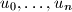$u_0, \ldots, u_n$ and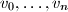$v_0, \ldots, v_n$ inductively by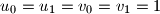$u_0 = u_1 = v_0 = v_1 = 1$, and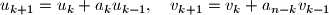for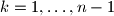$k = 1, \ldots, n - 1$.
Prove that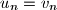$u_n = v_n$.
Izvor: France